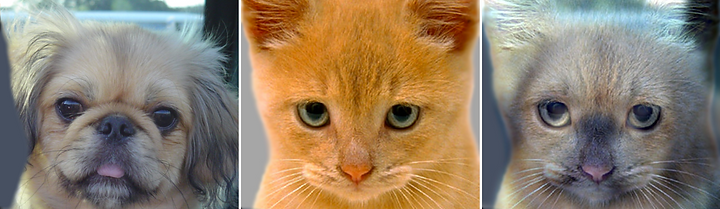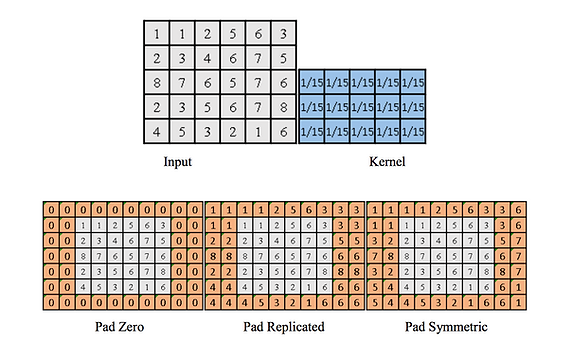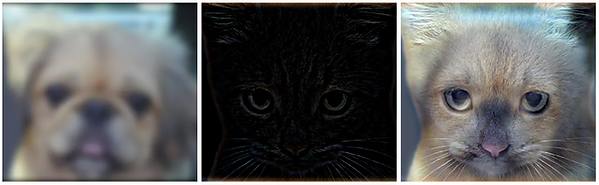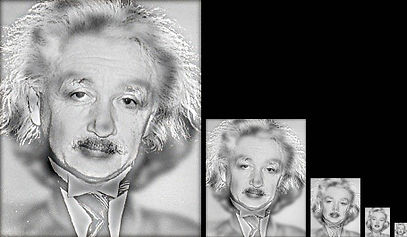top of page

# 2016

## Overview

The goal of this project is to write an image filtering function and use it to create hybrid images using a simplified version of the SIGGRAPH 2006 paper by Oliva, Torralba, and Schyns.

Hybrid images are static images that change in interpretation as a function of the viewing distance. The basic idea is that high frequency tends to dominate perception when it is available, but, at a distance, only the low frequency (smooth) part of the signal can be seen. By blending the high-frequency portion of one image with the low-frequency portion of another, we get a hybrid image that leads to different interpretations at different distances.

See the below examples:In this example, we can observe that the result hybrid image (the right one), looks like a combination of the two input images, a dog, and a cat. Yes, in fact, it's the sum of dog's low-frequency and cat high-frequency.

## Implementation

This project runs by Python code with OpenCV library. Numpy library is also needed for installation.

Noted that a high-frequency image can come from deducting low-frequency from the original image, so we only need to find the method to get the low-frequency image. And it is feasible by using the Gaussian filter, utilizing different padding methods. The result will blur the input image. Shown as below:

There is a free parameter, which can be tuned for each image pair, which controls how much high frequency to remove from the first image and how much low frequency to leave in the second image. This is called the "cutoff-frequency". In the paper it is suggested to use two cutoff frequencies (tuned for each image). In the skeleton code, the cutoff frequency is controlled by changing the standard deviation of the Gaussian filter used in constructing the hybrid images.

Below are the concept steps of implementations:

• Part 1 - Gaussian Filter by Padding

1. Read the two input images.

2. Set the cutoff frequency which is the to control the extent of the Gaussian filter.

3. Use OpenCV function "getGaussianKernel" to get the kernel, the size of the kernel is cutoff_frequency*4+1.

4. Since the above kernel is 1-dimension, to make it becomes 2-dimension, we should use OpenCV function "mulTransposed".

5. To get low-frequency image, we apply "my_imfilter" function, passing the image, kernel and the padding type as input.

6. In "my_imfilter" function, first split the image RGB channels into r,g,b variables separately.

7. Calculate the padding list width and height by using image width, image height, kernel width and kernel height.

1. After the padding, make the original r,g,b to be all 0.

2. Go through the padding list and calculate the Gaussian filter value of every pixel and store the value in r,g,b.

3. Merge r,g,b to get the filtered image, get the low -frequency image.

4. To get the high-frequency image, just use the original image subtract the low frequency of that image.

5. Add the low-frequency image and high-frequency image together, and get a Hybrid Image.## Resultsbottom of page